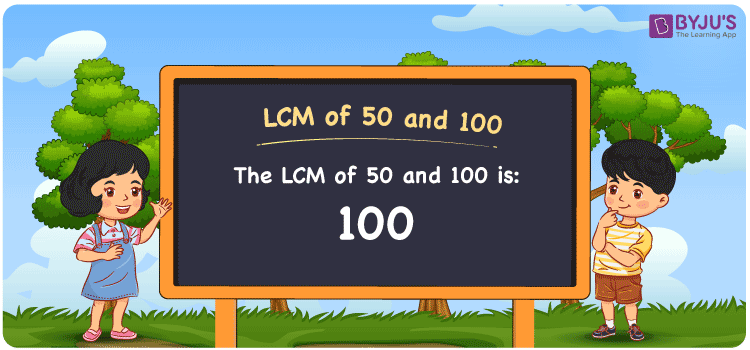# LCM of 50 and 100

LCM of 50 and 100 is 100. LCM stands for the Least Common multiple or Lowest Common Multiple. A number that is the smallest or the least and also that is divisible by the given set of numbers is known as the LCM. In the given set of numbers 50 and 100, 100 is the first number that is common in the set of multiples of 50 and 100. The LCM is also known as LCD, Least Common Divisor for 50 and 100 is 100. You can refer to LCM with Examples for detailed understanding.

## What is LCM of 50 and 100

The Least Common Multiple or Lowest Common Multiple of 50 and 100 is 100.## How to Find LCM of 50 and 100?

LCM of 50 and 100 can be determined using three methods:

• Prime Factorisation
• Division method
• Listing the multiples

### LCM of 50 and 100 Using Prime Factorisation Method

In the Prime Factorisation method for finding the LCM of 50 and 100, listing the product of factors that are prime to result 50 and also for 100. Then the universal set of products of factors forms the LCM.

50 = 2 × 5 × 5

100 = 2 × 2 × 5 × 5

LCM (50, 100) = 2 × 2 × 5 × 5 = 100

### LCM of 50 and 100 Using Division Method

In the Division Method, the given set of numbers are divided with the smallest number starting from 2 or other smaller prime numbers. The division is continued until 1 becomes the remainder.

 2 50 100 2 25 50 5 25 25 5 5 5 × 1 1

Therefore, LCM (50, 100) = 2 × 2 × 5 × 5 = 100

### LCM of 50 and 100 Using Listing the Multiples

By listing all the multiples of50 and 100, we can identify the smallest common multiple, which is the LCM. Below is the list of multiples for 50 and 100

 Multiples of 50 Multiples of 100 50 100 100 200 150 300

LCM (50, 100) = 100

## Video Lesson on Applications of LCM## Solved E×amples

What is the smallest number that is divisible by both 50 and 100?

Answer: 100 is the smallest number that is divisible by both 50 and 100.

What are the other common multiples of 50 and 100?

Answer: Other common multiples of 50 and 100 are 200, 300, 400, 500 … and so on.

## Frequently Asked Questions on LCM of 50 and 100

### What is the LCM of 50 and 100?

The LCM of 50 and 100 is 100.

### Is the LCM of 50 and 100 the same as the Highest Common Factor of 50 and 100?

No. LCM of 50 and 100 is 100 and the Highest Common Factor of 50 and 100 is 50.

### What is the common factor of 50 and 100?

1, 2, 5, 10, 25 and 50 are the common factors of 50 and 100.

### What are the first 3 common multiples of 50 and 100?

The first three common multiples of 50 and 100 are 100, 200, and 300.

### Is the LCM of 5 and 50 same as the LCM of 5 and 100?

No. LCM of 5 and 50 is 50, whereas the LCM of 5 and 100 is 100. They are not the same.